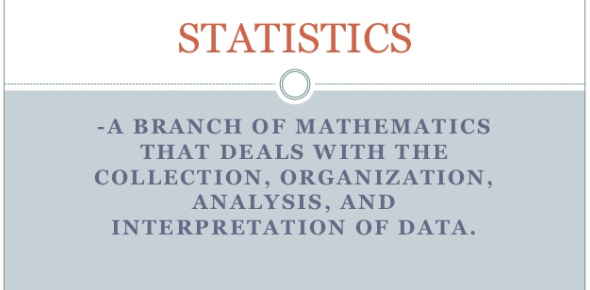# Ultimate Statistics Test! Trivia Quiz

15 Questions | Total Attempts: 155Settings.

Related Topics
• 1.
• A.

\$250 million

• B.

\$350 million

• C.

\$570 million

• D.

\$600 million

• 2.
• A.

0

• B.

6

• C.

8

• D.

10

• 3.
• A.

The tallest student in the class is a girl.

• B.

The shortest student in the class is a boy.

• C.

The range of the boys' heights is greater than the range of the girls' heights.

• D.

The median height of the girls is more than the median height of the boys.

• 4.
• A.

Mean

• B.

Median

• C.

Mode

• D.

Range

• 5.
According to the box-and-whisker plot shown below, what is the third quartile value?
• A.

100

• B.

90

• C.

80

• D.

70

• 6.
A retail store had total sales of \$436, \$650, \$530, \$500, \$650, \$489, and \$423.  Which measure of data would make the store's sales last week appear the most profitable?
• A.

Mean

• B.

Median

• C.

Mode

• D.

Range

• 7.
Hero earned the following scores on his science exams:  83, 88, 87, and 83.  If Hero earns a 90 on his next exam, which measure of tendency will give him the highest score?
• A.

Mean

• B.

Median

• C.

Mode

• D.

Range

• 8.
Mrs. Werner asked her 16 statistics students to write down an integer from 1 to 10.  To determine the most common number the students picked, which measure of central tendency should Mrs. Werner use?
• A.

Mean

• B.

Median

• C.

Mode

• D.

Range

• 9.
• A.

0

• B.

1

• C.

2

• D.

3

• 10.
Identify the type of graphical representation shown below.
• A.

Histogram

• B.

Bar graph

• C.

Line graph

• D.

Stem and leaf plot

• 11.
The manager of a sandwich shop is planning to make a circle graph showing the types of sandwiches sold in one day.  The table below summarizes the data. What central angle should the manage use for the section representing the ham-and-cheese sandwich?
• A.

270

• B.

75

• C.

54

• D.

15

• 12.
• A.
• B.
• C.
• D.
• 13.
• A.

Spirit ribbons earned \$342.

• B.

Homecoming photography earned \$161.50.

• C.

The bake sale earned \$237.50.

• D.

School logo mugs earned \$209.

• 14.
Identify the type of graphical representation shown below.
• A.

Line graph

• B.

Line plot

• C.

Stem and leaf plot

• D.

Box and whisker plot

• 15.
• A.

As the time spent practicing increases, the number of incorrect notes played remains constant.

• B.

The greater the time spent practicing, the fewer the number of incorrect notes played.

• C.

There is no relationship between the time spent practicing and the number of incorrect notes played.

• D.

The greater the time spent practicing, the greater the number of incorrect notes played.